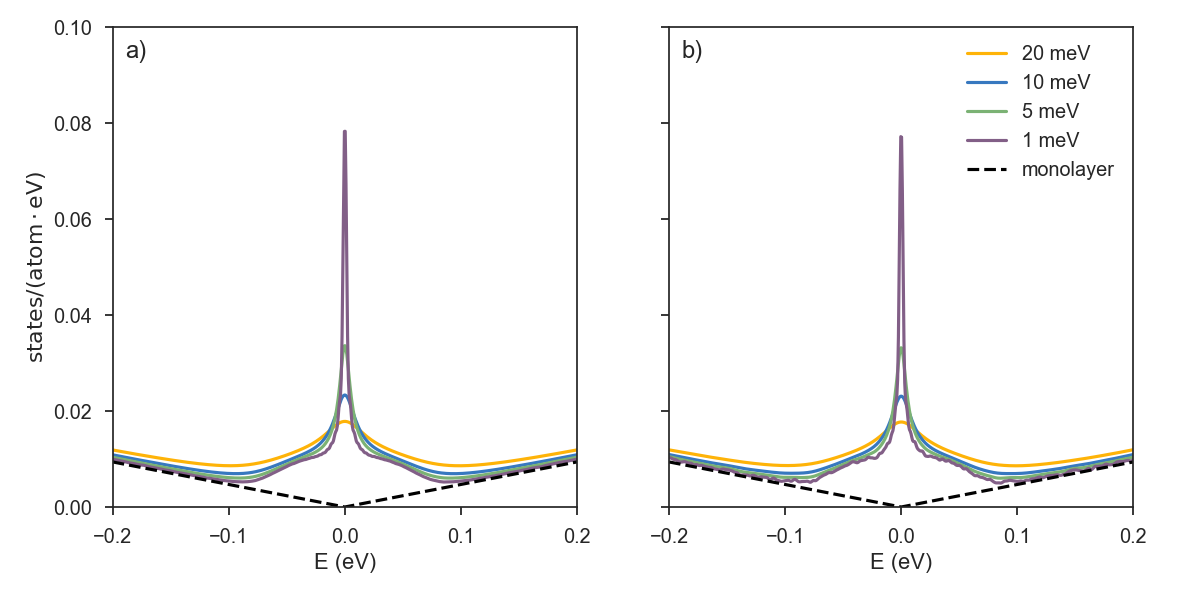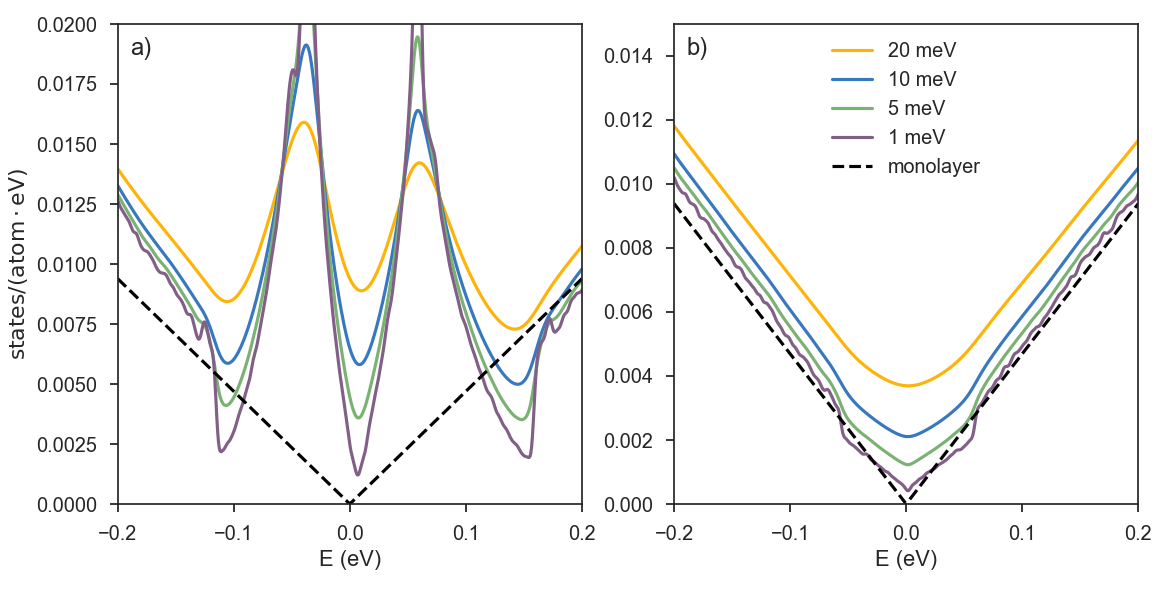# Large Systems

Up to this point, the idea of the tutorial was to show simple examples that could be reproduced on a desktop computer or a small work-station. But the purpose behind developing KITE was in building a flexible software for systems exceeding billion of atoms. Hence, in the following tutorial we will tackle the simulation of two different systems with their size being in the micrometer range.

### Graphene lattice with vacancy disorder¶

We simulated two graphene vacancy disordered systems, having more than 8.9 (large) and 0.5 (small) billion of atoms. The concentration of vacancy defects is 0.1%, while the number of moments used in the calculation is 15000. The two subplots show the zero energy modes in the density of state that are seen in graphene with diluted concentration of vacancies. The system in subplot (a) has size of l1 = 65536; l2=65536, while in subplot (b), l1 = 8064; l2=8192. The dashed curve is pristine graphene and solid curves stand for different resolutions: yellow (20 meV), blue (10 meV), green (5 meV), purple (1 meV).

There is an important difference in the two subplots that is related to the lattice size. The limiting factor for observing tiny details in the expanded spectral functions is the mean level spacing given by the system size. This can be seen when simulating a small lattice with high-resolution, as in the case of subplot (b) with 1 meV resolution: oscillations in the DOS are a sign that we are getting into the limit of showing the quantized nature of a finite size system in terms of discrete energy levels. Probing a small system with high resolution can result in non-physical effects. Working with high resolutions (and consequently large systems) is specially important when dealing with localization problems1.

If you would like to reproduce these results, we suggest you to check the script honeycomb_lat_vacancy.py under the examples. For the info, RAM requirements for running DOS on a small system specified above requires ~5GB and around 20 minutes on a node with 28 cores.### Moiré pattern¶

The second example is twisted bilayer graphene lattice in the clean limit, with the number of atoms exceeding ~0.7 billion. The model Hamiltonian _ of such a system has much larger coordination number (average number of neighbors per each atomic site), and the important parameter when estimating the running time (and the memory requirements) is the "effective" size, the product of the number of sites and the coordination number. In that sense, this system is in the mid-range, between the small and the large system of the previous example.

Depending on the rotation between adjacent layers, the resulting moiré pattern will have more or less effect on the low energy electrons in graphene. For high twist angles, van Hove singularities are far from the charge neutrality point. As the angle is decreased, they "move" to lower energies and eventually merge, forming flat bands at the Dirac point. This happens at so-called "magic" angles of rotation, which are of great interest recently, as they can provide an interesting playground to study correlations in graphene, with novel states of matter ranging from Mott insulators to superconductors.

Subplots a) and b) are showing the DOS for rotation angles of 2.005 (low) and 13.741 (high) degrees respectively. As in the previous case of disordered graphene, depending on the resolution, fine details around the Dirac point appear. High twist angles give rise to properties more similar to AB graphene. At low angles, the spectrum is much richer, and the linear spectrum of the two layers is fully changed due to the induced interlayer coupling. Apart from the electron-hole asymmetry, at higher energies, both densities follow the shape of the ones of a monolayer. Similar to the previous example, higher resolutions in the calculations lead to the appearance of oscillations in the density of state. Interestingly enough, as the angle is decreased, more states appear in the low energy spectrum, which reduces the mean level spacing. For the same high resolution of 1meV in subplot b) we can see the appearance of oscillations mentioned in the previous section, while the same DOS plot in subplot a) looks very smooth. When considering an arbitrary system, both spectrum and the size reflect on the level of details you are able to distinguish, while obtaining the results with physical meaning.Below, you can find a script used to configure the KITE model for twisted bilayer. The lattice of twisted bilayer graphene (especially at low rotation angles) has a much more complex unit cell compared to simple AB stacked bilayer, where for an arbitrary twisting one does not have the access to all the neighbors using simple neighboring distance vectors. More advanced search is needed for finding the unit cell connections. The search and the hopping calculation is done separately, because of our desire not to make this script too complex. Instead, we use an already defined lattice object that is loaded with a simple command:

    # define the angle
angle = 21.787 # or 13.174, 7.341, 2.005
# define the name of the pb.Lattice object
name = 'lattice_tblg_{:.3f}'.format(angle)